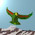## Saturday, October 8, 2011

### Neutrino Paper of the Day

From 2002:
Dynamical Electroweak Symmetry Breaking by a Neutrino Condensate
Stefan Antusch, Joern Kersten, Manfred Lindner, Michael Ratz

We show that the electroweak symmetry can be broken in a natural and phenomenologically acceptable way by a neutrino condensate. Therefore, we assume as particle content only the chiral fermions and gauge bosons of the Standard Model and in addition right-handed neutrinos. A fundamental Higgs field is absent. We assume instead that new interactions exist ...

1.This clearly refers to the same particle set as the extended Bilson-Thompson set.

2.One of the authors has more recently discussed a non zero $\theta_{13}$ in trimaximal mixing. They predict

$\theta_{13} = (\sqrt{2}/3)(m_2/m_3)$

in which we plug the Koide neutrino masses to obtain

$\theta_{13} = 0.083$

which is at the lower end of the T2K range. This has

$\sin^{2} \theta_{13} = 0.007$.

3.Doh, I mean

$\sin^{2} 2 \theta_{13} = 0.027$.

Note that the predicted $theta_{13}$ is very close to $1/12$.

Note: Only a member of this blog may post a comment.